﻿ 基于向量键合图法的3-PPR型平面并联机构动力学分析 The Dynamic Analysis for 3-PPR Planar Parallel Mechanism Based on Vector Bond Graph Method

Mechanical Engineering and Technology
Vol.05 No.03(2016), Article ID:18666,9 pages
10.12677/MET.2016.53028

The Dynamic Analysis for 3-PPR Planar Parallel Mechanism Based on Vector Bond Graph Method

Zhongshuang Wang, Hang Lv

School of Mechatronic Engineering, Qiqihar University, Qiqihar Heilongjiang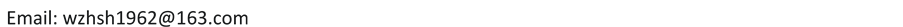Received: Sep. 6th, 2016; accepted: Sep. 25th, 2016; published: Sep. 30th, 2016ABSTRACT

For improving the reliability and efficiency of the dynamic analysis for planar parallel mechanism, the corresponding vector bond graph procedure is proposed. From the moving constraint relations between components of 3-PPR planar parallel mechanism, the corresponding vector bond graph model of the mechanism is made by assembling the vector bond graph models of planar rigid body, revolute joint and translational joint. For the problem of differential causality loop brought by nonlinear geometric constraints between components of the mechanism, the corresponding effective method is proposed. As a result, the algebraic difficulty in the mechanism automatic modeling and analysis is overcome. By the corresponding algorithm, the computer aided dynamic modeling and analysis of 3-PPR parallel mechanism is realized. By a practical example system, the reliability and validity of the procedure proposed are illustrated.

Keywords:3-PPR Planar Parallel Mechanism, Dynamic Modeling and Analysis, Vector Bond Graph, Causality, Joint Constraint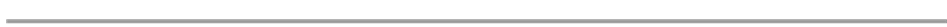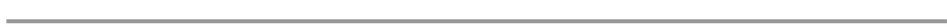1. 引言

3-PPR型平面并联机构是一种同时含有多个转动铰和移动铰的多自由度平面多体系统，相比全部为转动铰或含有少量移动铰的平面三自由度并联机构，其研究工作还远不够深入  。由于该类机构的拓扑结构及机构构件间的运动约束关系，应用向量键合图法对其进行动力学建模与分析，尚有若干理论及应用方面的问题需要解决。例如：如何建立该类平面并联机构的向量键合图模型；如何消除其向量键合图模型中贮能元件的微分因果关系，解决其给计算机辅助动力学建模所带来的代数困难，使其具有可计算性等，相关的文献报道甚少。本文基于向量键合图的基本概念，建立了具有可计算性的3-PPR型平面并联机构系统的向量键合图模型，实现了其计算机自动建模与动力学分析，有效地解决了上述问题。

2. 3-PPR型平面并联机构向量键合图模型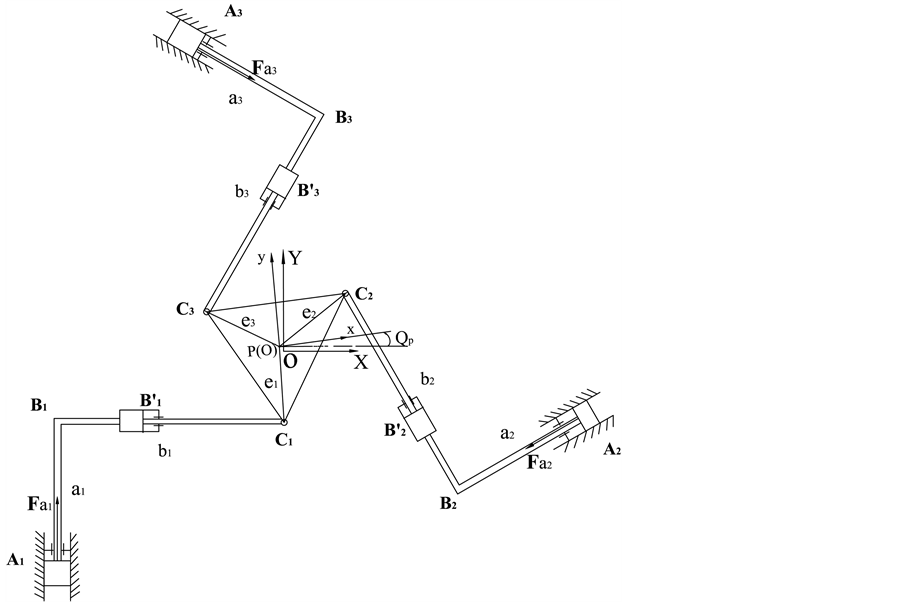Figure 1. The diagram of 3-PPR planar parallel mechanism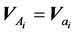(1)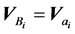(2)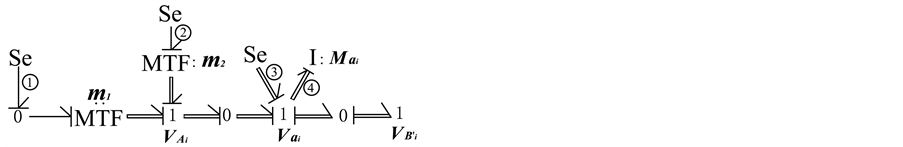Figure 2. The vector bond graph of component AiBi(3)Figure 3. The vector bond graph of component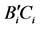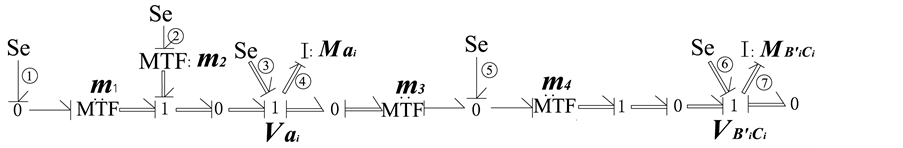Figure 4. The vector bond graph of moving chain i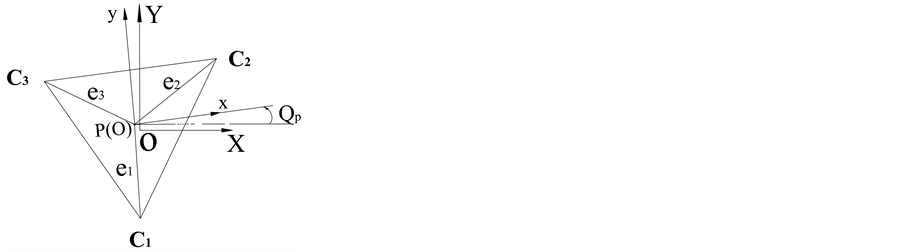Figure 5. Moving platform C 1 C 2 C 3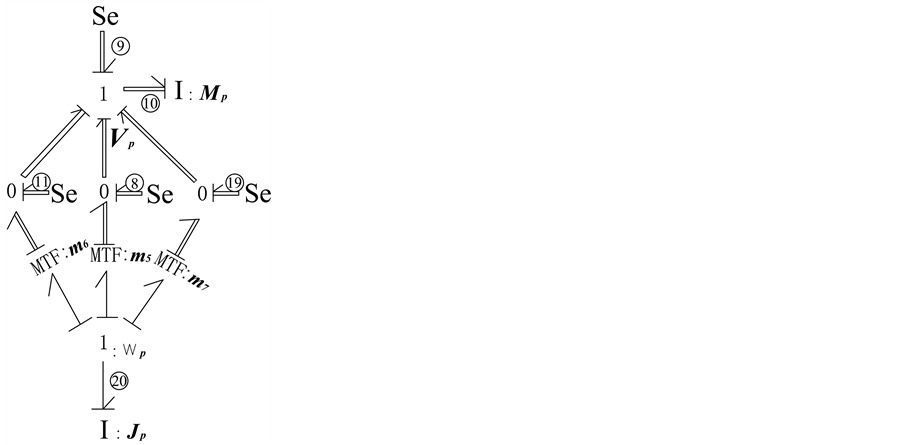Figure 6. The vector bond graph of moving platform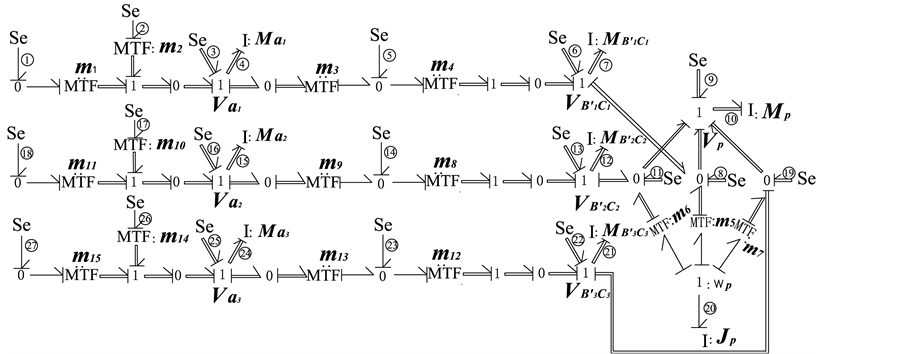Figure 7. The vector bond graph of 3-PPR planar parallel mechanism

3. 实际算例Figure 8. The driving force of component A3B3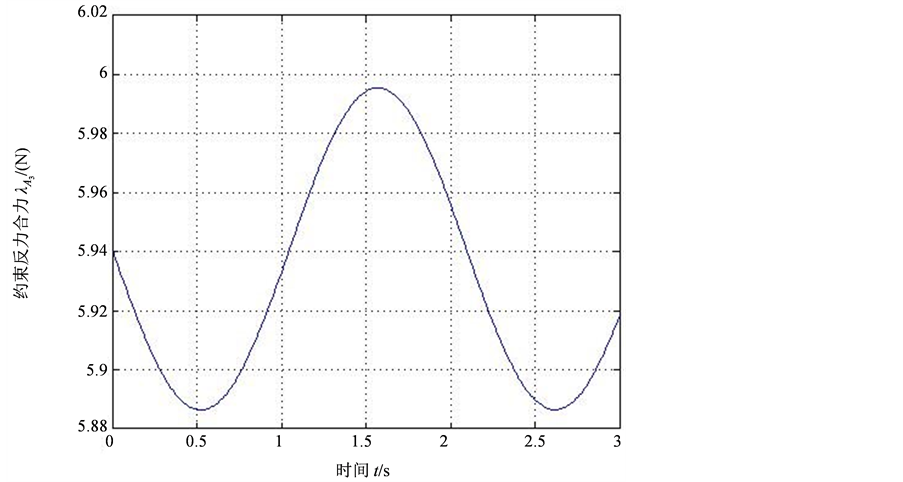Figure 9. The resultant constraint force of slip joint A3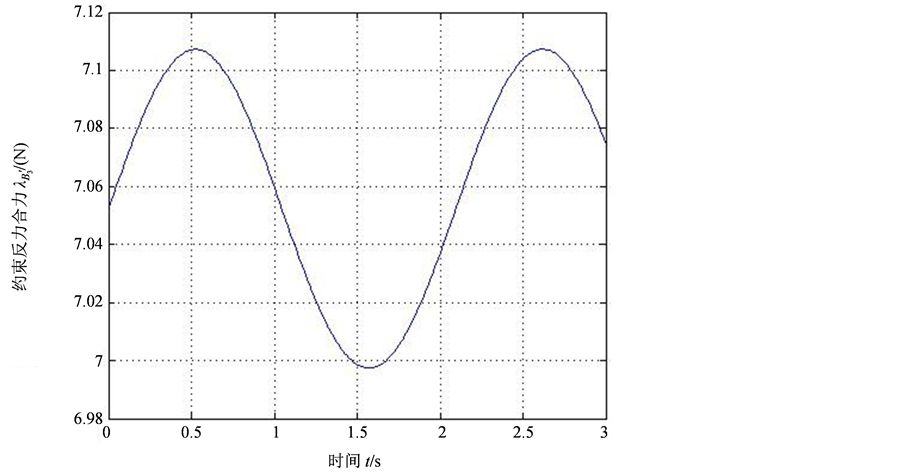Figure 10. The resultant constraint force of slip joint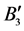Figure 11. The resultant constraint force of revolute joint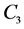4. 结论

The Dynamic Analysis for 3-PPR Planar Parallel Mechanism Based on Vector Bond Graph Method[J]. 机械工程与技术, 2016, 05(03): 238-246. http://dx.doi.org/10.12677/MET.2016.53028

1. 1. 黄真, 孔令富, 方跃法. 并联机器人机构学理论及控制[M]. 北京: 机械工业出版社, 1997: 1-11.

2. 2. 高晓雪. 3-PPR平面并联机构的运动学和动力学性能研究[D]: [硕士学位论文]. 太原: 中北大学机电工程学院, 2014.

3. 3. 郑相周, 唐国元, 编著. 机械系统虚拟样机技术[M]. 北京: 高等教育出版社, 2010: 1-9.

4. 4. Karnopp, D.C., Margolis, D.L. and Rosenberg, R.C. (2000) System Dynamics: Modeling and Simulation of Mechatronic Systems. Wiley, New York, 1-351.

5. 5. Kumar, A., Pathak, P.M. and Sukavanam, N. (2013) Trajectory Control of Two DOF Rigid-flexible Space Robot by A Virtual Space Vehicle. Robotics and Auto-nomous Systems, 61, 473-482. http://dx.doi.org/10.1016/j.robot.2013.01.004

6. 6. Cipek, M., Pavković, D. and Petrić, J. (2013) A Control-Orientied Simulation Model of Power-Split Hybrid Electric Vehicle. Application Energy, 101, 121-133. http://dx.doi.org/10.1016/j.apenergy.2012.07.006

7. 7. 王中双, 陆念力. 基于键合图的多体系统耦合动力学的发展及现状[J]. 中国机械工程, 2007, 18(17): 2137-2141.

8. 8. Behzadipour, S. and Khajepour, A. (2006) Causality in Vector Bond Graph and Its Application to Modelling of Multi-Body Dynamic Systems. Simulation. Modelling Practice and Theory, 14, 279-295. http://dx.doi.org/10.1016/j.simpat.2005.06.001

9. 9. 王中双, 王西峰. 混合驱动五杆机构动态静力分析向量键合图法[J]. 机械传动, 2016, 40(1): 77-81.